Study Guide

# Proofs

## Proofs

When detectives Benson and Stabler search a suspect's apartment, they aren't looking for just anything to give to the prosecutor. They need solid, tangible, and legally obtained evidence. (Although "legally obtained" is more of a suggestion than a requirement on Law and Order.) The point is that no jury or judge will convict based on a hunch or a guess. They'll need proof.

Think of proofs as arguments. If you're debating with your parents over your curfew and your main point is that you're responsible enough to stay out late, it's important to have reasons supporting this claim. Just an FYI, now wouldn't be a good time to bring up that flashing red light you ran a couple sections back.

The ability to support your statements with reasons is the essence of effective debate, and it's probably the most important skill to gain from studying geometry (and mathematics in general). It'll help you to be a more convincing speaker and might just get your curfew pushed back an hour or five.

• ### Formal Proofs

You have been cordially invited to attend the Formal Proof. Please dress in the appropriate attire. Form two columns and show this invitation at the door for admittance. Complimentary cheesecake will be provided.

Only one part of that is true and unfortunately, it's not the part about the cheesecake. We do have to form two columns. The left column is a (usually numbered) list of statements that logically follow each other. The right column is a list of reasons (or justifications) for these statements, just in case we need a little help going from one statement to the next.

Usually you're asked to prove an implication, a conditional statement with a hypothesis (sometimes several), and a conclusion. To prove such a thing, the first step is detaching the hypothesis from the conclusion, and assume the hypothesis as given. Then, write a bunch of statements and reasons until you end up with the conclusion.

Naturally, the tricky part is figuring out which statements will take you to the conclusion—it's a bit of a guessing game. Sometimes it'll take several tries before you reach the desired conclusion. Don't worry; when you finally get there, it'll be that much more satisfying.

### Sample Problem

Write a formal proof of the big train in the last section. That is, if A = B and B = C and C = D, then A = D.

Here, our given facts are the hypothesis of the conditional statement, namely A = B, B = C, and C = D. The fact we want to prove is A = D, so that should be our last statement in the list. Here's a good formal proof:

 Statements Reasons 1. A = B Given 2. B = C Given 3. C = D Given 4. A = C Transitive Property (1 and 2) 5. A = D Transitive Property (4 and 3)

We can list the given statements first, since they don't require any reason beyond "given." ("Given" simply means that you're assuming them as a hypothesis, so the problem "gives" them to you. Happy birthday!) Statement 4, A = C, follows from applying the transitive property to A = B and B = C.

Technically, we're also using the law of detachment here, but the laws of detachment and syllogism are used so often that we don't write down when we use them. In fact, almost every single non-given line of every proof uses one or the other; if we wrote them down, we'd spend hours on each proof just writing "detachment" twenty times. Instead, we'll say they're implied and save a couple (thousand) trees.

Finally, statement 5, A = D, follows from using the transitive property on statements 4 and 3. Since statement 5 is what we wanted to prove, we're done. Hooray!

Some formal or two-column proofs don't use the parentheses in the justification statements (telling exactly which of the statements you use), and sometimes it's even okay to lump together several steps at once. For example, some might consider this an acceptable proof:

 Statements Reasons 1. A = B, B = C, C = D Given 2. A = C Transitive Property 3. A = D Transitive Property

The idea is that we give enough information for the reader to be able to decipher our proofs. We think it's a good idea to write proofs more like the first one, since it's always a good habit to be as precise as possible, but how you write your proofs is up to you.

• ### Postulates and Theorems

Typically, the things in your reasons column will be one of the following: Given, some property of equality, a geometrical postulate (or axiom), or a theorem proved earlier.

We already know what "given" and the properties of equality are. What about the other stuff?

Postulates (also called axioms) are running assumptions about the objects we're talking about. For example, in geometry, we assume that exactly one line can be drawn between any two points. Obviously, whether that's true depends on how precisely we define the terms "line" and "point."

Rather than being bogged down by overcomplicated definitions, it's more convenient to just say, "Look, we all accept that there's only one line through these two points, so let's feel free to use it."

We all agree that there are 24 hours in a day. We could argue endlessly about how a day should be defined (when does a rotation of the earth begin and end?) and how hours came to exist, but all this bickering doesn't get us to school on time. At some point we simply declared that time is measured in hours after midnight, and that there are 24 hours in a day, and that's that.

Unfortunately, we got a bit carried away with these postulates and now there are time zones and daylight savings time, which we also have to deal with. Still, you can say, "Let's catch a 7:00 movie," and others will understand you. That's because they share the same common assumptions (or postulates) about how time is measured.

In Euclid's famous Elements, an example of ancient Greek geometry, he lists at the beginning 23 definitions and only 5 postulates. Instead of listing postulates all at once, we'll introduce them when they naturally come up. Also, be warned: there will be significantly more than 5.

Finally, a theorem is some fact that's already been proven. Since we've already shown it to be true, we can use it to show other things are true. This can be especially convenient when using the theorem saves you a lot of writing.

We can use this idea to build even longer trains.

### Sample Problem

Given: A = B, B = C, C = D, D = E.
Prove: A = E

 Statements Reasons 1. A = B Given 2. B = C Given 3. C = D Given 4. D = E Given 5. A = D Transitive Property (1, 2, and 3) 6. A = E Transitive Property (5 and 4)

Of course, theorems and postulates can be used in all kinds of proofs, not just formal ones. Paragraph or informal proofs lay out a logical argument in paragraph form, while indirect proofs assume the reverse of the given hypothesis to prove the desired conclusion. Proofs are like a bag of Bertie Bott's Every Flavor Beans: they might come in different (and sometimes awful-tasting) flavors, but they're all made of the same basic stuff. Except proofs are made of fact while Bertie Bott's are made of magic.

• ### Algebraic Proofs

Before diving headfirst into geometrical proofs, it's a good idea to revisit algebra. We've already learned how to solve equations for a variable. Now we'll do algebra in the format of the two-column proof.

### Sample Problem

Show that if 3x – 7 = 5, then x = 4.

Here, our given statement is 3x – 7 = 5, and we're asked to prove x = 4.

 Statements Reasons 1. 3x – 7 = 5 Given 2. 3x – 7 + 7 = 5 + 7 Addition of 7 to equation (1) 3. 3x + 0 = 5 + 7 Substitution of –7 + 7 = 0 into (2) 4. 3x = 5 + 7 Substitution of 3x + 0 = 3x into (3) 5. 3x = 12 Substitution of 5 + 7 = 12 into (4) 6. 3x⁄3 = 12⁄3 Dividing equation (5) by 3 7. x = 12⁄3 Substitution of 3x⁄3 = x into (6) 8. x = 4 Substitution of 12⁄3 = 4 into (7)

Is there such a thing as being too descriptive? Yep, and that was it, since over half the proof was devoted to telling the reader how to do arithmetic. We'll typically take numerical computation for granted, and write proofs like this:

 Statements Reasons 1. 3x – 7 = 5 Given 2. 3x = 12 Add 7 to both sides of equation (1) 3. x = 4 Divide equation (2) by 3

See? That proof looks a lot like how we'd write it in algebra. The only difference is that you give reasons as you go, convincing the readers (like your math teacher) that you know what you're doing. You got this.

### Sample Problem

Show that if 5(x + 12) = 30 and x + y = 100, then y = 106.

This time, our two given statements are 5(x + 12) = 30 and x + y = 100. We're supposed to prove y = 106. Here we go.

 Statements Reasons 1. 5(x + 12) = 30 Given 2. x + y = 100 Given 3. 5x + 60 = 30 Distributive property (1) 4. 5x = -30 Subtract 60 from both sides of (3) 5. x = -6 Divide both sides of (4) by 5 6. -6 + y = 100 Substitute x = -6 into (2) 7. y = 106 Add 6 to both sides of (6)

As you can see, there are lots of ways of phrasing your reasons. The important part is that you justify each step with why your statement is true. Of course, if your "reader" prefers it to be written a certain way, it's probably a good idea to follow his or her suggestions. Just saying.

• ### Geometric Proofs

One of the biggest differences between proofs in algebra and proofs in geometry is that geometrical proofs have pictures. By pictures, we mean images of geometric shapes, not lolcats.

Since geometry is concerned with things you can draw, like points, lines, angles, and the like, translating pictures into proofs and vice-versa can't really be avoided. That's okay, though. They're more interesting to look at than endless lines of text. Even this picture of a triangle gets your adrenaline pumping.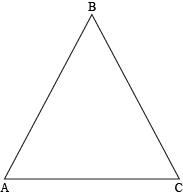ABC is made of three segments: AB, BC, and AC. Also, we can see that they make three angles: ∠BAC, ∠ABC, and ∠ACB. If that's already too much geometry lingo for you, get a quick refresher or a more in-depth look.

We can add little tick marks (be careful of Lyme disease!) to show that segments are congruent, which means they're exactly equal in measurement. For example, if we mark both AB and BC with a single tick mark like so: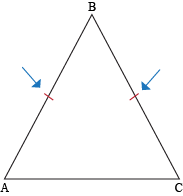It means that AB and BC are the same length, or ABBC. They're congruent (expressed by that equal sign with the squiggly on top). We can do the same thing with angles.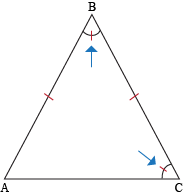Now ∠ABC and ∠ACB are marked congruent as well. By adding even more tick marks, we can account for several different congruencies.

### Sample Problem

Draw a figure with points P, Q, R, S and segments such that PQRS and QRPS.

First we draw the points and the segments: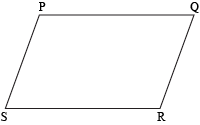Then we mark the first congruence with a single tick mark: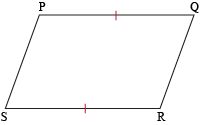Finally, we mark the second congruence, this time with double tick marks (so that we don't confuse all four segments as being congruent).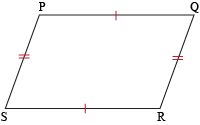In some instances, there are several ways to draw the same diagram. For example, if we placed the points like this: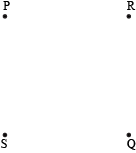and then drew the segments, we'd end up with some crossing segments.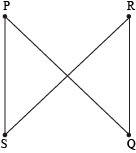There's nothing wrong with this picture, but it is a little harder to work with. For example, the next step is to put tick marks to show the congruence of PQ and RS.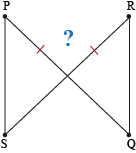It's hard to tell whether we're saying that the big segments are congruent or just the little parts before the intersection. In general, it's a good idea to try to draw diagrams so that no lines cross, if possible.

If they do cross, have no fear. Two crossing segments make vertical angles, like the ones here.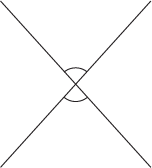Vertical angles are congruent, but it's not always necessary to draw their tick marks. It should be obvious from the image that they're opposite angles from an intersection of two segments.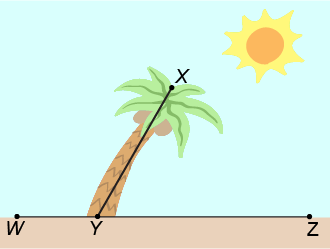When only part of a segment sticks out from the middle of a different one (like a leaning palm tree sticking out of the sand), the two angles are called supplementary. That means they both add up to 180°. We'll learn more about vertical and supplementary angles and all that good stuff later on. For now, just take our word for it.

• ### Congruence, Equality, and Geometry

One of the nice things about congruence is that it has a lot in common with equality. Since segments and angles are congruent when they have equal measures, it makes sense that congruence also has the reflexive, symmetric, and transitive properties.

In general, objects satisfying these three properties are called equivalence relations, since they behave a lot like actual equality. Martin Luther King Jr. would be proud.

So how can we take these congruent line segments and angles and convert them into proofs? Well, we'll show you.

### Sample Problem

Given the following figure, show that ∠1 is congruent to ∠3.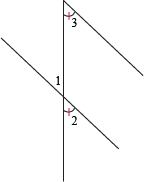Looking at the figure, we see that ∠1 and ∠2 are vertical angles, and ∠2 is congruent to ∠3 (by the tick marks). Thus, we can use those two facts as given statements.

 Statements Reasons 1. ∠1 and ∠2 are vertical Given (in figure) 2. ∠2 ≅ ∠3 Given (in figure) 3. ∠1 ≅ ∠2 Vertical angles are congruent (1) 4. ∠1 ≅ ∠3 Transitivity of congruence (3 and 2)

Like vertical angles, you can read "betweenness" directly from a diagram. For example, in the following picture,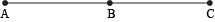we can assume B is between A and C, since the segment from A to C passes through B. Like in order to drive from L.A. to Seattle, we need to pass through Oregon. We don't really have a choice, but we might as well see Portland and Crater Lake. Make the best of it.

Remember that in this situation, AB + BC = AC. Seems obvious, right? This fact is often called the Segment Addition Postulate and frequently comes up in geometrical proofs.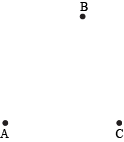Here, B may not be assumed between A and C because you have to go out of your way to pass through it (like passing through Des Moines on your way from L.A. to Seattle).

### Sample Problem

Given the diagram below, show that ACBD.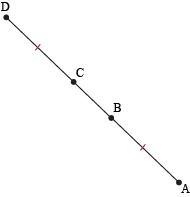From the diagram, we see that ABCD by the tick marks, so that's our given information.

This problem is quite a bit trickier than what we've covered so far, since we aren't explicitly given any information about the segments we want to prove something about. So…what do we do?

We can't jump straight to working with AC and BD. Instead, let's split them into more manageable pieces. AC can be split into AB and BC, while BD can be split into BC and CD. Right away we see that BC shows up in both of them, so we can match those parts up. This leaves AB and CD to match up. Whaddayaknow? Those are exactly the segments we're given information about.

If we write it out as a proof, this is what we have.

 Statements Reasons 1. AB ≅ CD Given (in figure) 2. AB = CD Definition of congruence (1) 3. AB + BC = BC + CD Addition of BC to (2) 4. AC = BD Segment addition postulate (3) 5. AC ≅ BD Definition of congruence (4)

It might seem like step 3 comes out of nowhere. Why are we adding this random BC to both sides of an equation? This is just a way to formally write out our intuitive argument about chopping big segments into smaller segments.

This idea of showing objects are congruent by splitting them into smaller pieces and matching them up is one of the most fascinating ideas in geometry. Aside from being the inspiration for several games and puzzles (like tangrams) and the backbone of many ancient proofs, this idea has led to some of the most breathtaking paradoxes in mathematics.

We've been focusing on segments for a while, and we think angles deserve some TLC, too. The Angle Addition Postulate states that the measures of two adjacent angles add up to the measure of the big angle they create. Sort of a no-brainer, but it's good to give it a name.

Some other definitions to note are: midpoints and bisectors split a segment into two congruent segments, and angle bisectors split an angle into two congruent angles. Supplementary angles add up to 180 degrees, and complementary angles add up to 90 degrees.

Again, don't worry too much about the nuts and bolts of all this. We promise we won't lead you astray.

### Sample Problem

Show that if two angles are complementary to the same angle, then they're congruent.

We'll name the angles ∠1, ∠2, and ∠3. Original, we know. They're just easier to spell than names like "Bartholomew" and "Cornelius."

Given: ∠1 and ∠3 are complementary, ∠2 and ∠3 are complementary.
Prove: ∠1 ≅ ∠2

Before writing our formal proof, let's take a look at what we have. We know m∠1 + m∠3 = 90, and also that m∠2 + m∠3 = 90. Since we want to know information about m∠1 and m∠2, we can solve the two given equations for m∠1 and m∠2. This gives us m∠1 = 90 – m∠3 and m∠2 = 90 – m∠3. Now we're getting somewhere! The right sides of those equations are the same. We can now formalize this.

 Statements Reasons 1. ∠1 and ∠3 are complementary Given 2. ∠2 and ∠3 are complementary Given 3. m∠1 + m∠3 = 90 Definition of complementary angles 4. m∠2 + m∠3 = 90 Definition of complementary angles 5. m∠1 = 90 – m∠3 Subtract m∠3 from (3) 6. m∠2 = 90 – m∠3 Subtract m∠3 from (4) 7. m∠1 = m∠2 Transitive property of equality (5 and 6) 8. ∠1 ≅ ∠2 Definition of congruence (7)